## Algebra

Showing posts with label LCD. Show all posts
Showing posts with label LCD. Show all posts

### Complex Rational Expressions

It turns out that we have all the tools necessary to simplify complex algebraic fractions.

Rational Expressions and Equations Playlist on YouTube

The numerator and denominator of these rational expressions contain fractions and look very intimidating.  We will outline two methods for simplifying them.

Method 1: Obtain a common denominator for the numerator and denominator, multiply by the reciprocal of the denominator, then factor and cancel if possible.Method 2: Multiply the numerator and denominator of the complex fraction by the LCD of all the simple fractions then factor and cancel if possible.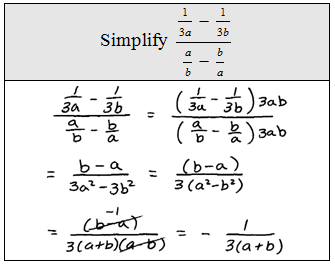To illustrate what happened after we multiplied by the LCD we could add an extra step.For the following solved problems, both methods are used. Choose whichever method feels most comfortable for you.

Simplify using method 1. Simplify using method 2.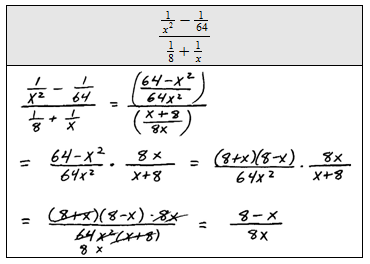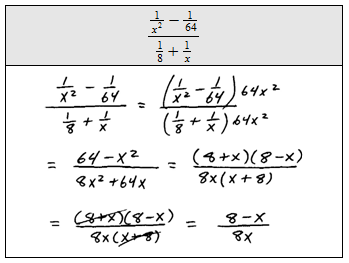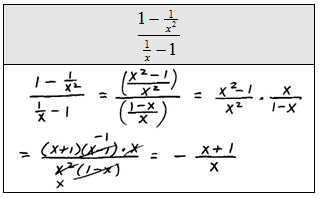### Elementary Algebra Exam #4

Click on the 10 question exam covering topics in chapter 7 (Rational Expressions and Equations). Give yourself one hour to try all of the problems and then come back and check your answers.

Simplify (Assume all denominators are nonzero.)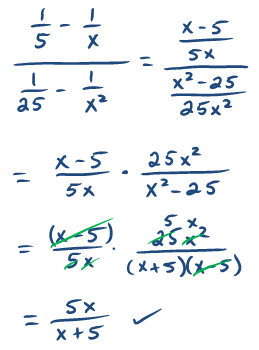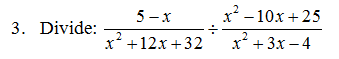Perform the operations and state the restrictions.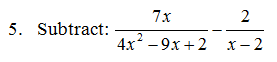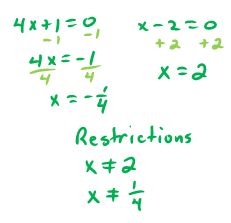Solve.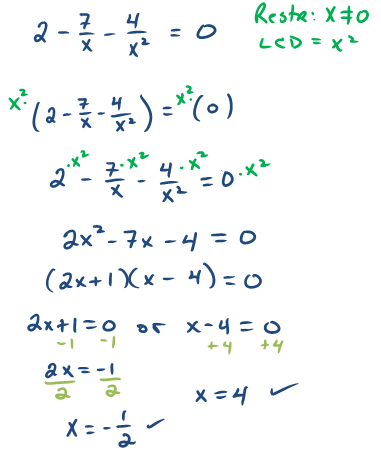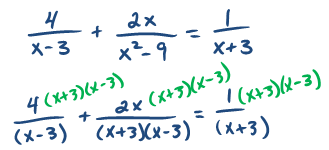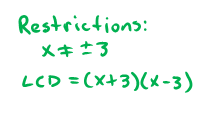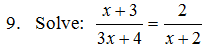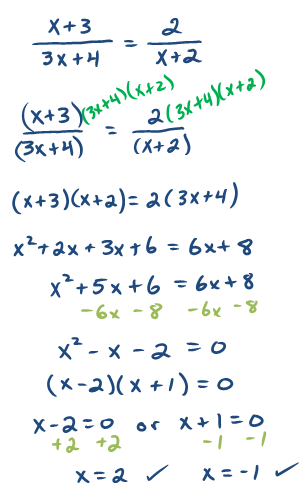10. The sum of the reciprocals of two consecutive odd integers is 4/3.  Set up an algebraic equation and use it to find the two integers.---